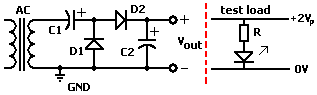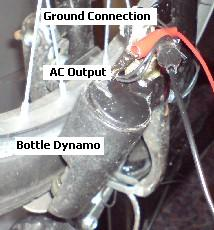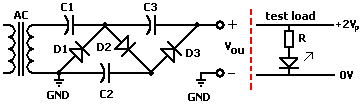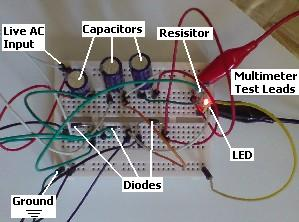# Making Voltage Doublers and Multipliers

A voltage doubler or voltage multiplier is a circuit easily made from a few components which enables the voltage from an AC power source to be increased. This is particularly useful in situations where the load current is low, but a higher voltage than is provided by the AC source is required. In addition these circuits rectify the AC volage to DC votlage which can be used to power DC loads and charge batteries.

A voltage tripler for example can be used to increase the voltage from a 6V rated Sturmey Archer Dynohub (or other bicycle dynamo hubs) so that it can be used to charge a 12V Battery, or to do the same for a low voltage stepper motor wind turbine.

In this article we will put together and test a couple of basic voltage multipler circuits. Links to online resources explaining exactly how voltage multiplier circuits work are provided at the end of the article for anyone who wants to find out more.

### Voltage Doubler Circuit

To make a voltage doubler circuit only two capacitors and two diodes are required costing pennies each. The circuit diagram is displayed below:### Testing the Voltage Doubler

A bicycle bottle dynamo was used as the AC power source (see photo below). Since this is used to power two incandescent light bulbs, it should certainly have enough power to provide 10-20mA of current to the LED we planned to use as the test load.First the AC voltage of the dynamo was measured directly with a multimeter. Spinning the front wheel of the bicycle as quickly as possible by hand, the maximum voltage reading recorded was 5.04 Volts AC.

Putting this AC output voltage through a bridge rectifier, the maximum DC voltage was measured at 5.12 Volts DC. It is this DC voltage we are interested in seeing increase using our voltage doubler circuit since the doubler will also act as a rectifier converting the AC voltage output by the dynamo into DC voltage.

The voltage doubler was built using prototyping breadboard as per the circuit diagram provided earlier in this article. The wheel was spun rapidly by hand ten times and an average taken of the maximum voltages recorded from each spin. This average was 10.12 Volts DC – almost double that recorded using the bridge rectifier – showing that the voltage doubler (5.12V to 10.12V) circuit was working.

### Voltage Tripler

A voltage tripler or other larger voltage multiplier can be built simply by extending the voltage doubler circuit with the addition of extra capacitors and diodes. One extra capacitor and one extra diode are required for each increase in multiple – therefore a tripler has a total of three capacitors and three diodes as shown below.The circuit above was built again on prototyping breadboard, the wheel spun ten times, and the average maximum voltage recorded to be 13.64 Volts DC. Not quite triple, but that could be due to the increased amount of voltage dropped across the diodes in the circuit. Another alternative is that the LED was using all of the available current and emptying the third capacitor C3 before it could reach the predicted 15 VDC. (Remember that every increase in voltage is matched by a fall in current since the overall maximum power output of the dynamo is fixed. See Ohm’s Law)As a final test, the 510 Ohm resistor (in series with the LED) was replaced with a 1,000 Ohm resistor to halve the current reaching the LED to see why the voltage tripler circuit was falling short. The experiment was repeated and the new average maximum voltage recorded was 14.84 Volts.

Therefore the voltage tripler circuit was working perfectly – the problem was insufficient power from the small bottle dynamo reaching the LED. Had a larger dynamo been used a voltage of around 15 Volts DC would have been recorded first time.

### Voltage Multipliers

Getting more multiples of voltage is simply a matter of adding more capacitors and diodes to the existing tripler circuit until the required voltage is reached. As further multiples are added the voltage rating of the capacitors used must be considered – for example in a voltage quadrupler circuit, the fourth capacitor would receive 20V, so 16V-rated capactitors could not be used. In addition the power of the AC source must be considered to calculate if enough current at the increased voltage will be available for the load.

### Increasing Efficiency of Voltage Multipliers – Diode Choice

The forward voltage drop in a standard silicon diode such as the 1N4001 we used in our experiments is 0.6-0.7 Volts. This is not insignificant, and the accumulated drops in voltage make a real difference to the final output voltage from the multiplier as power is wasted (as dissipated heat) from the diodes. To increase efficiency, it is better to use Germanium diodes, or easier to find Schottky Diodes as these have a voltage drop of just 0.25-0.4V, halving the power lost in the diodes compared to silicon diodes and therefore increasing the output voltage.

### Find out More About Voltage Multipliers

In this article we have not covered the sometimes complicated theory involved in voltage multipliers. If you are interested in finding out more then there are numerous online resources of which a few are listed below:

Voltage Multipliers from play-hookey.com.
Basic Multiplier Circuits – introduces the Villard cascade (as seen in the voltage tripler above) and discusses the practical limitations of voltage multipliers .
Voltage doubler, tripler, and quadrupler circuits – from coolcircuit.com.

And here is a video showing a voltage quadrupler in action together with the circuit diagram, and a time lapse showing its construction.